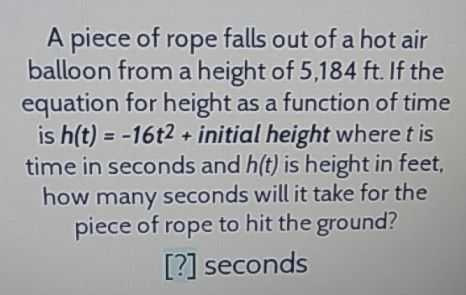### ¿Todavía tienes preguntas de matemáticas?

Pregunte a nuestros tutores expertos
Algebra
PreguntaA piece of rope falls out of a hot air balloon from a height of $$5,184 ft$$ . If the equation for height as a function of time is $$h ( t ) = - 16 t ^ { 2 } +$$ initial height where $$t$$ is time in seconds and $$h ( t )$$ is height in feet, how many seconds will it take for the piece of rope to hit the ground?

[?] seconds